##### Basic Math & Pre-Algebra For Dummies Book + Workbook Bundle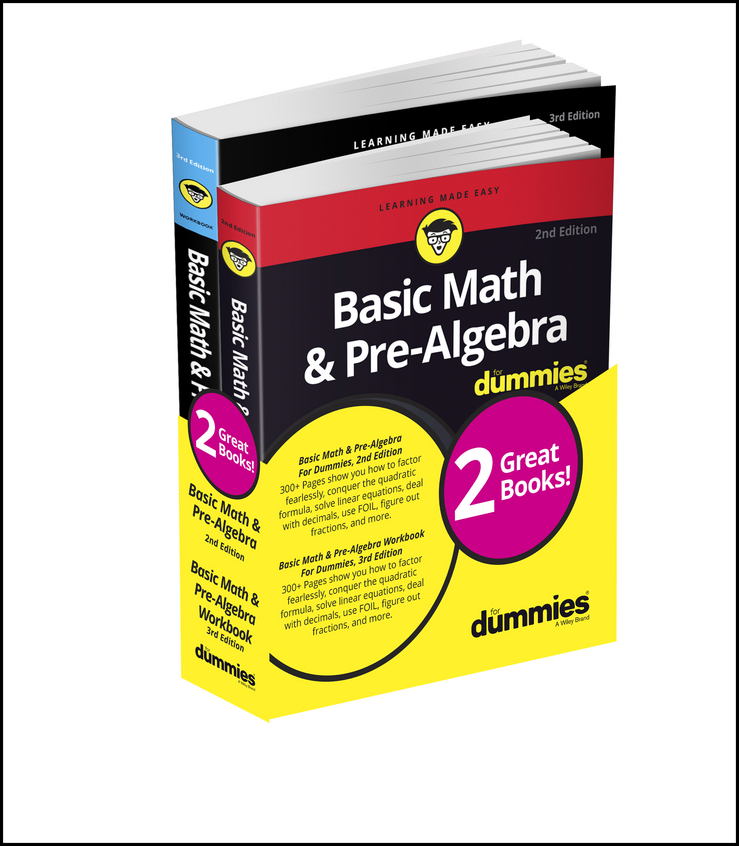Dividing fractions is as easy as pie! In fact, after you change mixed numbers to improper fractions, dividing fractions is just like multiplying fractions, except that you flip the numerator and the denominator of the second fraction.

Example: You bought 6 1/2 pounds of sirloin steak, and you want to cut it into pieces that weigh 3/4 pound each. How many pieces will you have?

First, change the word problem into an equation, which looks like this: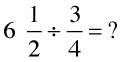1. Change all mixed numbers to improper fractions.

The only mixed number is 6 1/2, so multiply the denominator by the whole number (2 × 6 = 12), and then add that result to the numerator (12 + 1 = 13). Now the problem looks like this: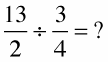2. Invert (flip) the second fraction, placing the bottom number on top and the top number on the bottom; then change the division symbol to a multiplication symbol.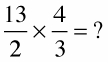3. Multiply the fractions, and reduce if necessary.

Multiply the numerators, straight across the top of the fraction line (13 × 4 = 52). And then multiply the denominators straight across the bottom (2 × 3 = 6).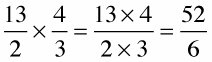Always change your answers to mixed numbers and reduce them, if possible.

In this case, to change the mixed number to an improper fraction, you need to divide 52 by 6. Doing so gives you 8 with 4 remaining. So 8 is the whole number and you place the remainder (4) over the denominator (6) for an answer of 8 4/6. 8 4/6 can be reduced further because 4 and 6 are divisible by 2. After you divide the numerator and denominator by 2, your answer becomes 8 2/3.

Here's how it looks: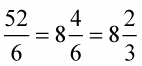You end up with 8 hefty steaks that weigh 3/4 pound each (or 12 ounces), and one steak that weighs 8 ounces (2/3 of 12).